Next: An algebraic characterization of Up: Generalization to the n-var Previous: Polytope Convexity and Extreme

#### The Fundamental Theorem of Linear Programming

Having established all the necessary concepts and properties of the solution space of n-var LP's, we are now ready to discuss the Fundamental Theorem of Linear Programming. This theorem can be stated as follows: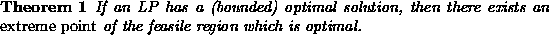Proof (Sketch): We establish the validity of Theorem 1, through a series of observations:

1. First notice that according to the previous discussion, the feasible region of an LP is a polytope, and thus, convex.
2. Furthermore, since we assume that the LP has an optimal solution, let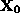denote such an optimal point. The optimal objective value will be denoted by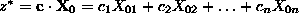.
3. Then notice that pointcannot be interior to a line segment that is not perpendicular to the direction of improvement to the ``isoprofit'' hyperplanes - w.l.o.g., let's assume a maximization LP for our discussion - defined by vector. Otherwise, by moving on this line segment in the direction of improvement of the ``isoprofit'' hyperplanes,we would be able to obtain another point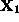of the feasible region, such that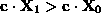. But this contradicts the assumption thatis an optimal point. Figure 9 depicts this argument.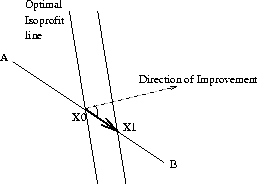Figure 9: Why an optimal solution to an LP cannot be interior to a line segment not perpendicular to the direction of improvement of the "isoprofit" hyperplanes

4. However, pointcan be interior to a line segment of the feasible solution space which is perpendicular to the direction of improvement of the optimal ``isoprofit'' hyperplane. This, in fact, corresponds to a situation of many optimal solutions. In this case, notice that this line segment must have at least one enddefined by the fact that one (or more) additional constraint is binding at this point. Otherwise, the problem is ill-posed, since we can vary some variable(s) at will over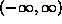with this variation affecting neither the constraints nor the objective.
5. Hence,, being on the optimal ``isoprofit'' hyperplane, is another optimal point at which an additional constraint is binding. Then, there are two possibilities: (i)is an extreme point of the feasible region, in which case we are done, or (ii)is interior point to another line segment lying in the optimal ``isoprofit'' hyperplane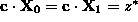, which binds, however, an additional constraint, compared to point. In this case, repeating the argument above, we establish the existence of another end point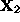, determined by the binding of at least one more constraint. Then, we repeat the entire argument for, and so on. Figure 10 depicts this part of the proof.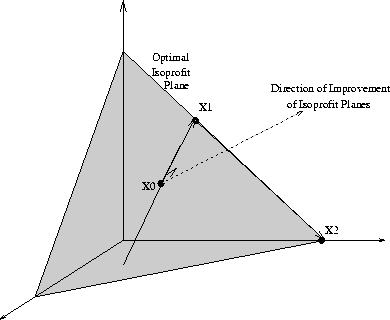Figure 10: Identifying an optimal extreme point on the optimal isoprofit hyperplane

6. Finally, notice that every time we bind an additional constraint, we restrict the (sub-)space of optimal solutions considered by one ``degree of freedom''. Since an n-dim space has n ``degrees of freedom'', the number of end points visited in the argument above before we find one that it is an extreme point is finite. Thus, this last observation establishes the existence of an optimal extreme point for the case of many optimal solutions, and the proof is complete.

The discourse of the previous proof has also revealed a very important property of extreme points: At these points, the number of binding constraints is such that it allows zero ``degrees of freedom'', or, in other words, these constraints define the point uniquely. Starting from this observation, in the next section we provide a series of algebraic characterizations of the extreme points, which will eventually allow us to analytically manipulate the set of extreme points of an LP, in the context of the Simplex algorithm. As it has been previously mentioned, this algorithm exploits the result stated in the Fundamental Theorem above, by limiting the search for an optimal solution over the set of extreme points of the polytope defining the LP feasible region. In the next section, we shall show that this set is finite and discrete, so it can even be exhaustively enumerated. Simplex algorithm provides an efficient way to search this set.Next: An algebraic characterization of Up: Generalization to the n-var Previous: Polytope Convexity and Extreme

UAL Data
Fri Jun 20 15:03:05 CDT 1997